# Exponential equations

## Supporting facts

Any increasing (decreasing) function between each acquires its value only in one point of this period.When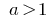the exponential function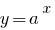is increasing.

When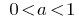the exponential functioncomes in.

When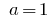the exponential functionwas.

The decision model equations one must know the properties of roots and degrees.

## Examples of solution of simple exponential equations

1.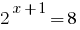2. Solution: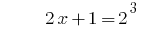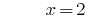Answer: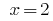3.4. Solution: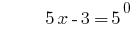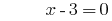Answer: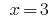5.6. Solution:

No roots (so)

7.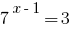8. Solution: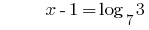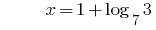Answer: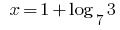## Examples of the solution of model equations by reduction to the simplest

______________________________________________________________________

If the left and right parts of the equations are illustrative only of the work, fractions, roots AO extent, it is advisable using basic formulas to try to record both parts of the equation as powers of one base.

______________________________________________________________________

### Example 1:

Rozwarte equations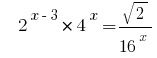.

Solutions: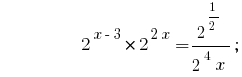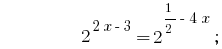Answer:.

______________________________________________________________________

If one part of the exponential equation is a number and the other containing all the members of the expression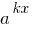(exponents differ only by free members), it is convenient in this part of the equation to put aside the smallest degree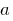.

______________________________________________________________________

### Example 2:

Rozwarte equations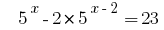.

Solutions: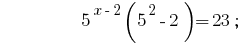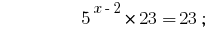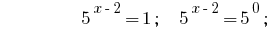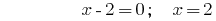Answer: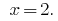.

## Examples of more complex exponential equations

______________________________________________________________________

Get rid of numeric terms in the exponents (using right-to-left basic properties of degrees).

If possible, reduce all degrees to one basis and perform a change of variables.

______________________________________________________________________

### Example 3:

Rozwarte equations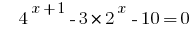.

Solutions: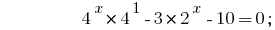Given that, we reduce the degree by one base 2: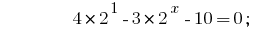Substitution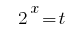gives the equation:Backward substitution gives the equation, where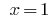or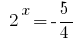- no roots.

Answer: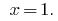______________________________________________________________________

If not degree can be reduced to one basis, try to reduce all degrees to two bases so as to obtain a homogeneous equation.

______________________________________________________________________

### Example 4:

Rozwarte equations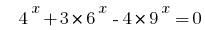.

Solutions:

Let's give all the extent to the two bases 2 and 3: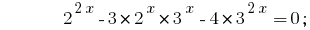Have a homogeneous equation. For its solution divide both sides by;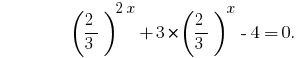Substitution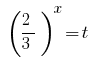gives the equation: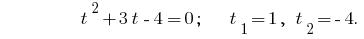Backward substitution gives the equation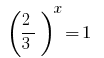, whereor- no roots.

Answer: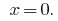______________________________________________________________________

In other cases, we transfer all the terms in the equation into one part and try to decompose the obtained expression into factors, or apply special techniques to the solution in which we use properties of the corresponding functions.

______________________________________________________________________

### Example 5:

Rozwarte equations.

Solutions:

If in pairs to group members in the left side of the equation, and in each pair stand out a common factor, we get :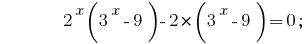Make the brackets a common factor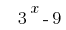:Then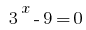or.We get two equations 1)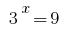where2)where.

Answer: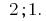Tags:
Chapter:
Versions in other languages:
Share with friends: